## Fundamental Relationship between Averages

Time-average , delayed-average , moving-average and delayed-moving-average have several interesting relationships.

## Relationship of Time-Average and Moving-averageWhen we set the length of kernel equal to the length of the sequence, that is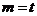, the moving-average is equal to the time-average (tag=movag)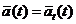(13)

Proof: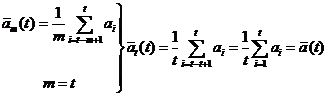QED

## Relationship of Delayed-Average and Delayed-Moving-average

If we set the kernel length of delayed-moving-average into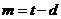, the delayed-average is equal to the delayed-moving average (dag=demovag)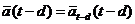(14)Proof: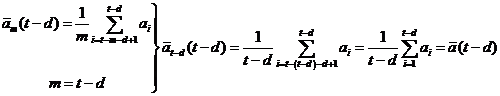QED

## Relationship of Delayed-Average and Moving-average and Time-Average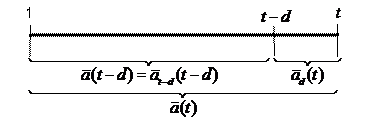From the average decomposition diagram (ADD), we can decompose the average of whole sequence into two parts of weighted averages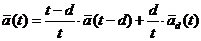(15)

I call Equation (15) as the fundamental theorem of average : time-average is equal to the sum of length-weighted delayed-average and moving-average.

Proof:

Multiply both side of equation (15) with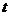we have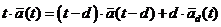(15a)

Expanding the right hand side of equation (15a) we have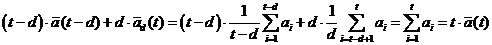QED.

From equation (15) we can derive what I call as Shift Property of Average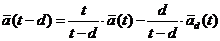(16)

Notice that the right hand side of (16) is evaluated for timewhile the left hand side is evaluated at time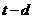Based on equation (15), we can also derive a ratio of difference. The ratio between the difference of moving average to delayed average and the difference of average to delayed average is equal to the ratio between the delay and the length of sequence in consideration. (relationship of movag, dag and tag)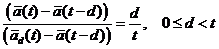(17)

Proof:

Taking the difference of average and delayed-average multiplies by its own length, we have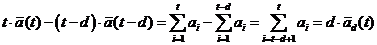because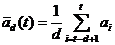.

Expand the second term in the left hand side and put the right hand side to the left we get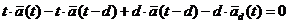Gather the same terms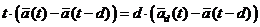Then,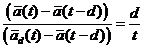QED

## Relationship of Delayed-Average and Delayed-Moving-Average and Time-Average

We can also decompose the measurement sequence into three parts of length-weighted averages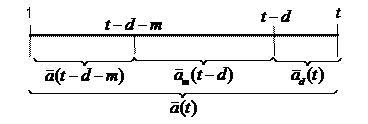From the average decomposition diagram (ADD), we can decompose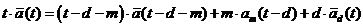(18)

Combining equation (15) and (18) we have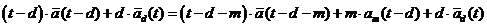Removing redundant last term of both side gives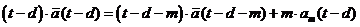(19)

Equation (19) is the relationship of delayed-average, time average and delayed moving average